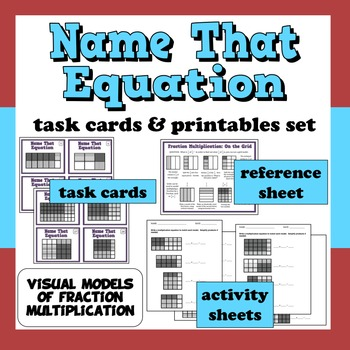# Name That Equation - fraction multiplication task cards + printables set4th - 6th
Subjects
Resource Type
Formats Included
• PDF
Pages
16 pages

### Description

Help your kids build a stronger understanding of visual representations of fraction multiplication with this set of task cards & printables. The Common Core Standards for Mathematics place a premium on using visual representations of numeric relationships, as well as having students understand the relationship between visual representations and numeric equations. The task cards and activity sheets in this set are the perfect tool for helping your students become familiar with the relationship between visual representations of fraction multiplication and corresponding multiplication equations.

Included:
• graphic reference sheet
• 2 two-page activity sheets (key included)

The printables consist of a graphic reference sheet and two different two-page worksheets. The graphic reference sheet shows how grids can be used to model finding a fraction of a fraction and multiplying a fraction by a fraction. Before you have your students complete the cards, you can have them glue the reference sheet in their journals. When I give students sheets of this size, I sometimes have them fold it in half and glue it across two pages of the journal, allowing it to fold open when they open their journal; alternatively, they may fold it in half and glue one half down on one journal page, folding open the sheet when they need to read it. Your students can use the journal insert as a guide while they work on the cards, as well as when they complete other tasks that relate to multiplying a fraction by a fraction.

The 32 cards present students with different models of fraction multiplication. For each card, students must write a multiplication equation to match the model presented. The cards increase in difficulty, with later cards using more complex fractions as factors, and some of the models will require your students to simplify the products in their equations.

The two provided worksheets can be used to evaluate your students’ ability to relate numeric equations to models of fraction multiplication. The worksheets are formatted similarly, though the models on each are different. You can use these activity pages in a variety of ways. You could give one as a pre-test, then teach your lesson and allow students to practice with the task cards, and then give the second worksheet as an independent post-test. You could also have the students work on the task cards, then complete one of the worksheet as guided practice with yourself, a partner, or a small group, and then give the second worksheet as an independent assessment. The worksheets could also be given as homework, center assignments, or any other purpose that fits your teaching style or classroom routines.

I hope your students enjoy these resources and are able to build their understanding of fraction multiplication. – Dennis McDonald
Total Pages
16 pages
Included
Teaching Duration
N/A
Report this Resource to TpT
Reported resources will be reviewed by our team. Report this resource to let us know if this resource violates TpT’s content guidelines.